Latest Banking jobs   »

# Quantitative Aptitude Quiz For LIC ADO Mains 2023- 16th April

Q1. Nikhilesh invested certain amount in three different schemes A, B and C with the rate of interest 10 percent per annum 12 percent per annum and 15 percent per annum respectively. If the total interest accrued in one year was Rs. 3200 and the amount invested in scheme C was 150% of the amount invested in scheme A and 240% of the amount invested in scheme B, what was the amount invested in scheme B?
(a) Rs. 8000
(b) Rs. 5000
(c) Rs. 6500
(d) Rs. 12000
(e) Rs. 10000

Q2. A, B and C invest in the ratio of 3 : 4 : 5. The percentage of return on their investments are in the ratio of 6 : 5 : 4. Find the total earnings, if B earns Rs. 250 more than A :
(a) Rs. 6000
(b) Rs. 7250
(c) Rs. 5000
(d) Rs. 7000
(e) Rs. 8000

Q3. Rajeev earns 3/2 times in January, April, July and October than his average earning of Rs. 600 per month in the rest of the month. So his savings in the January, April, July and October goes to 5/4 times that of the rest months saving of Rs. 400 per month in the year. The average expenditure of per month is:
(a) Rs. 266.67
(b) Rs. 250
(c) Rs. 233.33
(d) Rs. 433.33
(e) Rs. 245

Q4. If a man walks at the rate of 5 kmph, he misses a train by 7 minutes. However, if he walks at the rate of 6 kmph, he reaches the station 5 minutes before the arrival of the trair. Find the distance covered by him to reach the station.
(a) 4 km
(b) 6 km
(c) 5 km
(d) 7 km
(e) 9 km

Q5. 25% of the employees of department A and 16% of the employees of department B took part in a function. Participants from department A were 500 in number but 252 employees of department B did not take part in the function. Find the total no. of employees in A and B together?
(a) 2200
(b) 2300
(c) 2000
(d) 2500
(e) 2400

Q6. The edge of the cube is 10 cm. Given E is the center of the semicircle and it is mid-point of the diagonal of the given cube.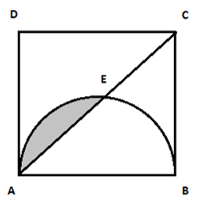Quantity I: Area of the shaded region.
Quantity II: 10 cm2.
(a) Quantity I > Quantity II
(b) Quantity I < Quantity II

(c) Quantity I ≥ Quantity II

(d) Quantity I ≤ Quantity II

(e) Quantity I = Quantity II or No relation

Q7. The length of a passenger train moving at a speed of 45km/h is 250m. The length of a Rajdhani train is 750m which can move at a maximum speed of 135km/h.
Quantity I: Time taken by the passenger train to cross a person standing on the platform.
Quantity II: Time taken by the passenger train to cross the Rajdhani train coming from opposite direction.
(a) Quantity I > Quantity II
(b) Quantity I < Quantity II
(c) Quantity I ≥ Quantity II
(d) Quantity I ≤ Quantity II
(e) Quantity I = Quantity II or No relation

Q8. There are 3 vessels A, B and C full of mixture of milk and water. Vessel A contains 5 liters of water and 25 liters of milk, Vessel B contains 15 liters of water and 30 liters of milk and Vessel C contains water and milk in the ratio 1 : 5. 20%, 40% and 30% of the total mixtures from vessels A, B and C respectively is taken and poured into a fourth vessel. The ratio of milk and water in the fourth vessel is 16 : 5.
Quantity I: Capacity of vessel C in litres.
Quantity II: 80 litres.
(a) Quantity I > Quantity II
(b) Quantity I < Quantity II
(c) Quantity I ≥ Quantity II
(d) Quantity I ≤ Quantity II
(e) Quantity I = Quantity II or No relation

Q9. P, Q and R can complete a piece of work in 8, 12 and 24 days respectively. They work on alternate days.
Quantity I: Time taken by them to complete the work if P works on day 1, Q works on day 2 and R works on day 3 and so on.
Quantity II: Time taken by them to complete the work if Q works on day 1, R works on day 2 and P works on day 3 and so on.
(a) Quantity I > Quantity II
(b) Quantity I < Quantity II
(c) Quantity I ≥ Quantity II
(d) Quantity I ≤ Quantity II
(e) Quantity I = Quantity II or No relation

Q10. There are two bags A and B. Bag A contains 5 red, 3 green and 4 blue balls while Bag B contains 8 blue, 4 green and 6 red balls.
Quantity I: Probability of getting at most 1 red ball if 3 balls are drawn at random from Bag B.
Quantity II: Probability of getting at least 2 red balls if 3 balls are drawn at random from Bag A.
(a) Quantity I > Quantity II
(b) Quantity I < Quantity II
(c) Quantity I ≥ Quantity II
(d) Quantity I ≤ Quantity II
(e) Quantity I = Quantity II or No relationL1Difficulty 3

Directions (11 -15): Given below pie chart shows percentage distribution of total meetings held in four quarters of 2016, while table shows percentage of finance meetings held in these four quarters. Read the data carefully and answer the questions.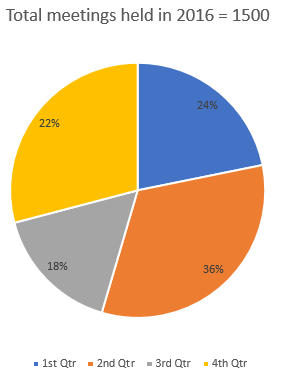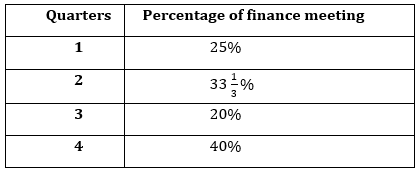Note – Three (Finance, Management and Expenditure) types of meeting held in each of quarter.

Q11. If total management meetings held in 3rd quarter is 40% more than total expenditure meetings held in that quarter and total management meetings held in 4th quarter is 20% more than total Expenditure meetings held in that quarter, then find difference between total management meetings held in 3rd & 4th quarter?
(a) 14
(b) 18
(c) 16
(d) 12
(e) 20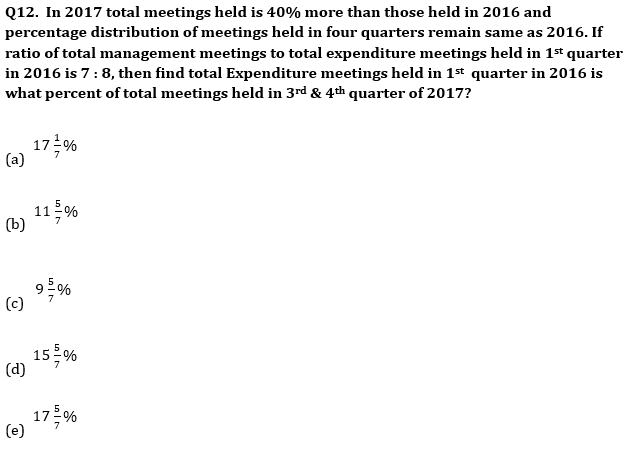Q13. If total expenditure meetings held in 2nd quarter is 40% less than that of total management meetings held in that quarter, then find total expenditure meetings held in 2nd quarter is what percent less than total management & expenditure meetings held in 1st quarter?
(a) 40%
(b) 45%
(c) 25%
(d) 60%
(e) 50%

Q14. If total meetings held in 1st quarter of 2017 is 25% more than total finance meeting held in 1st & 3rd quarter of 2016 together and ratio of finance meeting, management meeting and expenditure meeting held in 1st quarter of 2017 is 9 : 7 : 5, then find ratio of total management meeting held in 1st quarter of 2017 to total finance meeting held in 2nd quarter of 2016?
(a) 1: 4
(b) 1 : 2
(c) 1 : 5
(d) 1 : 3
(e) None of these

Q15. Total management meetings held in 1st , 2nd & 4th quarter is 25%, 40% & 20% more than total expenditure meetings held in these quarter respectively. If total management & expenditure meetings held in 3rd quarter is equal, then find average number of management meetings held in these four quarters of 2016?
(a) 142
(b) 152
(c) 144
(d) 148
(e) 140

Solutions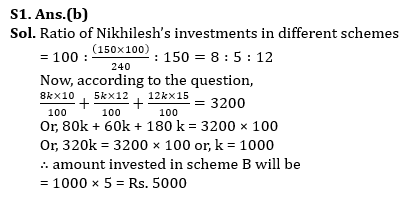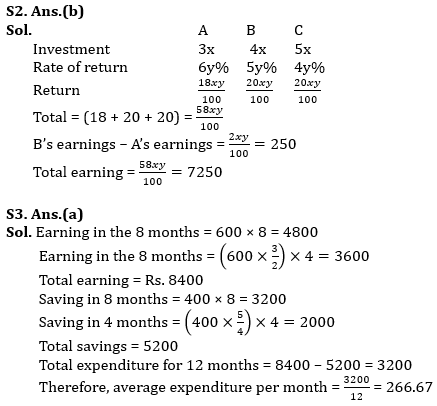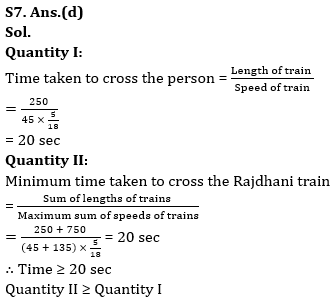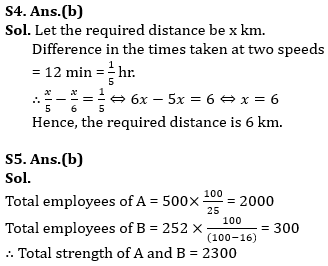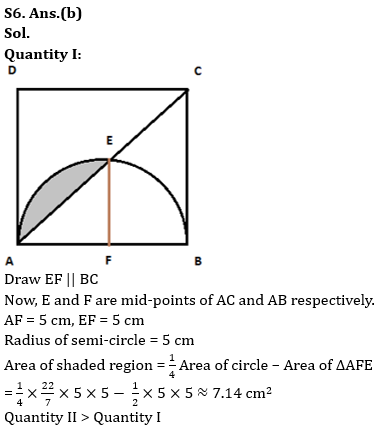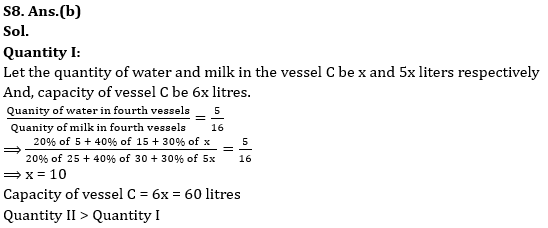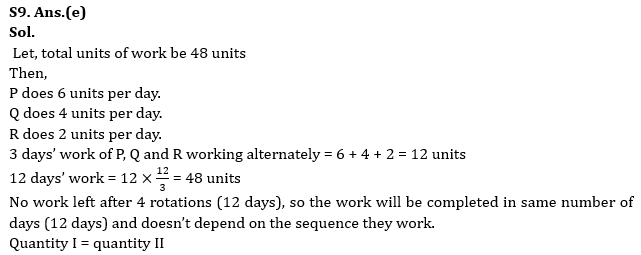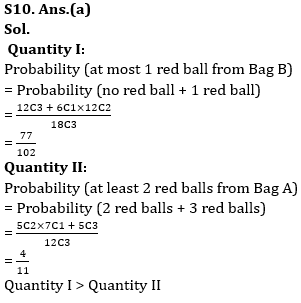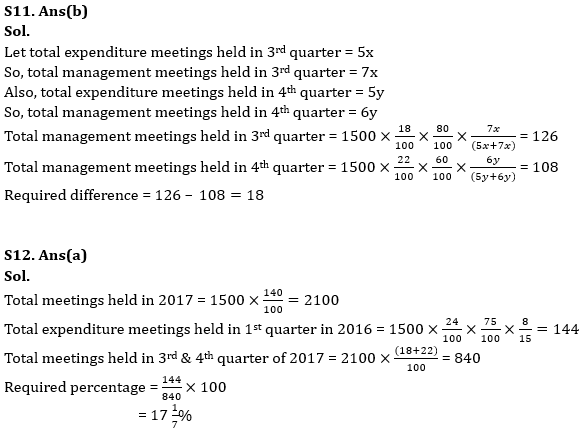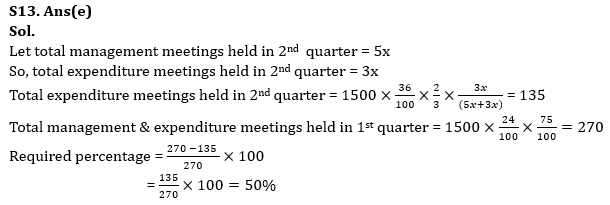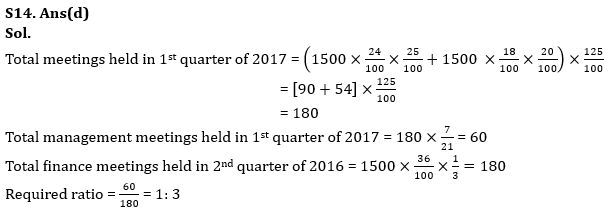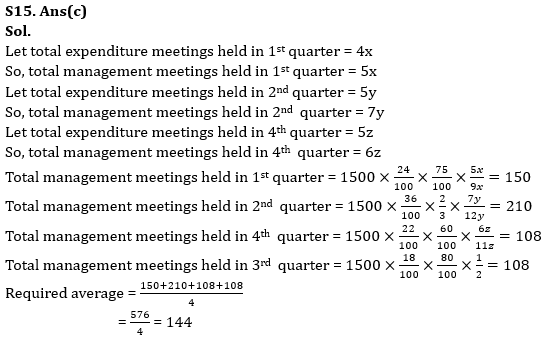## FAQs

### When is the LIC ADO Mains Exam scheduled?

The LIC ADO Mains exam is scheduled to be held on 23 April 2023

#### Congratulations!Union Budget 2023-24: Free PDF